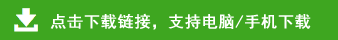# 苏教版三年级数学下册期中测试卷（无答案）

1.直接写出得数。（每题0.5分，共8分）
20×40=        24×3=         60×50=        15×4=
10×24=        73×10=        76×0=         23×3=
60+90=         500×8=        3×900=        72-28=
30×30=        23+48=         22×4=         660÷6=
2.用竖式计算，打*的要验算。（3+4+3，共10分）
25×16=             *79×38=             5×680=

3.计算下列各题。（每题3分，共9分）
（800-254）÷6          96÷3×8           38+62×4

1.32×79的积是（    ）位数，大约是（    ）。
2.与59相邻的两个数的乘积是（    ）。
3.在（  ）里填上合适的单位。